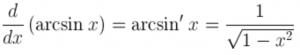# Derivative of arcsin(x)

What is the derivative of the arcsine function of x?

The derivative of the arcsine function of x is equal to 1 divided by the square root of (1-x2):